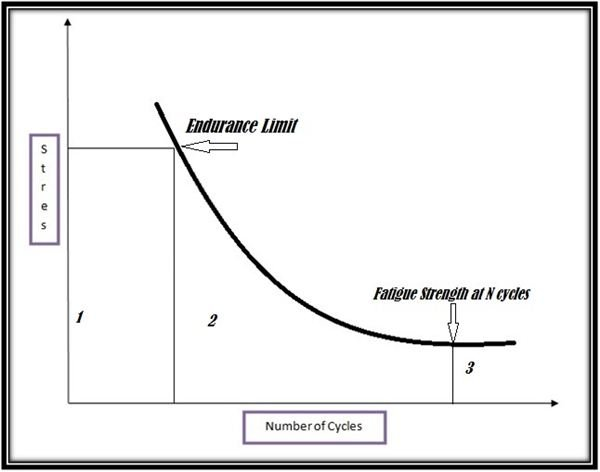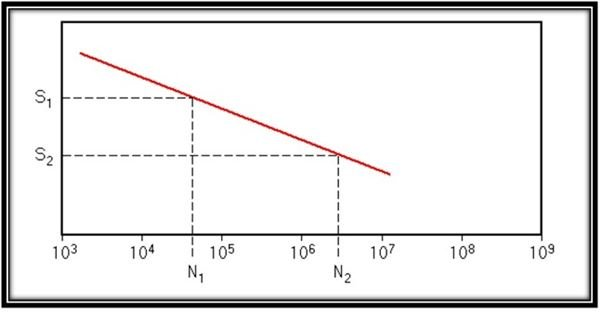# S-N Fatigue Curves for Shear Stress

S-N curves are two-dimensional curves, plotting cycles of failure (N) and shear stress (S) on X-Y axis on a logarithmic scale. The prerequisite of plotting these curves is that the stress has to be cyclic in nature. Every material, when subjected to alternate or cyclic loads, will fail if the loading surpasses its resistance. However, if rupture occurs at a load less than the projected resistance of the material, then it will be termed as fatigue. Major factors affecting the resisting force of a material, known as the "endurance limit," include:

1. Corrosive environment
2. Temperature variations.
3. Surface finish and exposed area.
4. Notches.

The main reason behind fatigue rupture is the development of minor cracks due to successive loading. With every loading, these cracks grow bigger, resulting in untimely failure. Wöhler curves help in the determination of upper limit of shearing stress that can be exerted on a material.

## Plotting the Wöhler Curves

Plotting S-N curve includes plotting initial strain against life to failure. In the laboratory, a sinusoidal stress is applied and the process is known as the Coupon Testing process. These curves are also known as a Stress-Life diagram. For example, let us consider fatigue behavior of compression members like springs. The following figure has been divided into three different regions.Region 1 denotes the area of ogilocyclic fatigue. In this region, rupture occurs at very small alternations and the material is often subjected to plastic deformations.

Region 2 denotes the area of limited endurance.

Region 3 is known as the security zone under low constraint.

Now let us consider an ideal S-N curve, which would be a straight line. By approximating a stress curve, we can calculate the value of stress amplitude. The curve is used to determine the Basquin Slope.N1 = N2 (S1 / S2)1/b is the general relationship between two failure cycles at different time intervals.

and the Basquin Slope is determined by the equation:

b = -[(logS1 – logS2)/ (log N2 – logN1)], where

S1, S2 are stress values for corresponding number of failure cycles denoted by N1 and N2. b denotes slope of the S-N curve.

Once slope of the curve is determined, it becomes very easy to find out the value of stress amplitude for any two S-N values on the curve.

Wöhler Curves can also be used to determine the values of fatigue ratio for different materials. Fatigue Limit can be defined as ratio of ultimate strength (Su) and endurance limit (Se) of a material. The value of fatigue limit usually varies between 0.25 and 0.60.

For instance, steel's fatigue ratio is expressed as Se = 0.55 Su.

Notches mean discontinuity on the surface of structural members and notches play an important role in deciding the stress values for materials under testing. These discontinuities are also known as stress concentration factors. Stress concentration factor of a structural member is calculated as Kt= (Smax/S), where Smax is maximum local stress and S is nominal stress of the member.With the help of Kt, we can determine the fatigue notch factor using the S-N fatigue curves for shear stress. The fatigue notch factor relates the

un-notched fatigue strength, which is the endurance limit for ferrous members like steel, of a member to its notched fatigue strength:

Kf = Se(un-notched) / Se(notched), Se(un-notched) is achieved only under ideal conditions.

## Applications of Wöhler Curves

Wöhler Curves have a wide scope of use. They are used mainly in the construction sector. Other than that, they are used in the aerospace industry, heavy lifting cranes, bridging and shipping, railway industry, offshore drilling and petrochemical industry. S-N curves are very helpful for design engineers because predicting safe loads that a structure or building can bear is very important for safe designing. Different codes and regulations mark it as a top priority to make sure structural components are able to bear cyclic loads. There is a Modified Wöhler Curve Method, which is used to determine joint strengths. Welded joints riveted joints, made of steel and aluminum can be brought under the test and with the help of Wöhler Curve, safe design shear stress can be predicted. While designing, Wöhler Curve values are not used as such and 10-15% margin of safety is taken into account.

## References

Stress Life Diagram, https://www.ux.uis.no/~hirpa/KdB/ME/S-N%20diagram.pdf

Modified Wöhler Curve Method, https://www.sciencedirect.com/science/article/pii/S1350630708001052

Images : Created by the author